Search by Topic

Resources tagged with Generalising similar to Three by Three:

Filter by: Content type:
Age range:
Challenge level:

There are 90 results

Broad Topics > Using, Applying and Reasoning about Mathematics > Generalising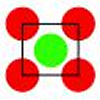Centred Squares

Age 7 to 11 Challenge Level:

This challenge, written for the Young Mathematicians' Award, invites you to explore 'centred squares'.Button-up Some More

Age 7 to 11 Challenge Level:

How many ways can you find to do up all four buttons on my coat? How about if I had five buttons? Six ...?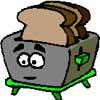Broken Toaster

Age 7 to 11 Short Challenge Level:

Only one side of a two-slice toaster is working. What is the quickest way to toast both sides of three slices of bread?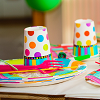Sitting Round the Party Tables

Age 5 to 11 Challenge Level:

Sweets are given out to party-goers in a particular way. Investigate the total number of sweets received by people sitting in different positions.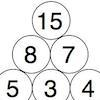Build it up More

Age 7 to 11 Challenge Level:

This task follows on from Build it Up and takes the ideas into three dimensions!Sums and Differences 2

Age 7 to 11 Challenge Level:

Find the sum and difference between a pair of two-digit numbers. Now find the sum and difference between the sum and difference! What happens?Build it Up

Age 7 to 11 Challenge Level:

Can you find all the ways to get 15 at the top of this triangle of numbers? Many opportunities to work in different ways.Sums and Differences 1

Age 7 to 11 Challenge Level:

This challenge focuses on finding the sum and difference of pairs of two-digit numbers.Tiling

Age 7 to 11 Challenge Level:

An investigation that gives you the opportunity to make and justify predictions.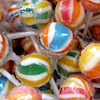Lots of Lollies

Age 5 to 7 Challenge Level:

Frances and Rishi were given a bag of lollies. They shared them out evenly and had one left over. How many lollies could there have been in the bag?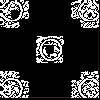Doplication

Age 7 to 11 Challenge Level:

We can arrange dots in a similar way to the 5 on a dice and they usually sit quite well into a rectangular shape. How many altogether in this 3 by 5? What happens for other sizes?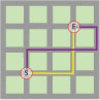The Add and Take-away Path

Age 5 to 7 Challenge Level:

Two children made up a game as they walked along the garden paths. Can you find out their scores? Can you find some paths of your own?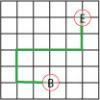Journeys in Numberland

Age 7 to 11 Challenge Level:

Tom and Ben visited Numberland. Use the maps to work out the number of points each of their routes scores.Calendar Calculations

Age 7 to 11 Challenge Level:

Try adding together the dates of all the days in one week. Now multiply the first date by 7 and add 21. Can you explain what happens?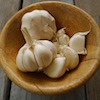Growing Garlic

Age 5 to 7 Challenge Level:

Ben and his mum are planting garlic. Use the interactivity to help you find out how many cloves of garlic they might have had.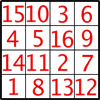Magic Constants

Age 7 to 11 Challenge Level:

In a Magic Square all the rows, columns and diagonals add to the 'Magic Constant'. How would you change the magic constant of this square?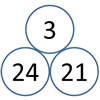Unit Differences

Age 5 to 7 Challenge Level:

This challenge is about finding the difference between numbers which have the same tens digit.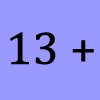What Could it Be?

Age 5 to 7 Challenge Level:

In this calculation, the box represents a missing digit. What could the digit be? What would the solution be in each case?Bundles of Cubes

Age 7 to 11 Challenge Level:

Watch this animation. What do you notice? What happens when you try more or fewer cubes in a bundle?Age 5 to 11 Challenge Level:

Try out this number trick. What happens with different starting numbers? What do you notice?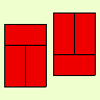Fault-free Rectangles

Age 7 to 11 Challenge Level:

Find out what a "fault-free" rectangle is and try to make some of your own.Round the Three Dice

Age 7 to 11 Challenge Level:

What happens when you round these three-digit numbers to the nearest 100?Number Differences

Age 7 to 11 Challenge Level:

Place the numbers from 1 to 9 in the squares below so that the difference between joined squares is odd. How many different ways can you do this?Simple Train Journeys

Age 5 to 11 Challenge Level:

How many different journeys could you make if you were going to visit four stations in this network? How about if there were five stations? Can you predict the number of journeys for seven stations?Round the Two Dice

Age 5 to 7 Challenge Level:

This activity focuses on rounding to the nearest 10.Round and Round the Circle

Age 7 to 11 Challenge Level:

What happens if you join every second point on this circle? How about every third point? Try with different steps and see if you can predict what will happen.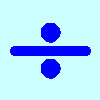Division Rules

Age 7 to 11 Challenge Level:

This challenge encourages you to explore dividing a three-digit number by a single-digit number.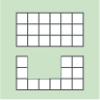Area and Perimeter

Age 7 to 11 Challenge Level:

What can you say about these shapes? This problem challenges you to create shapes with different areas and perimeters.Dice Stairs

Age 7 to 11 Challenge Level:

Can you make dice stairs using the rules stated? How do you know you have all the possible stairs?Oddly

Age 7 to 11 Challenge Level:

Find the sum of all three-digit numbers each of whose digits is odd.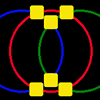Magic Circles

Age 7 to 11 Challenge Level:

Put the numbers 1, 2, 3, 4, 5, 6 into the squares so that the numbers on each circle add up to the same amount. Can you find the rule for giving another set of six numbers?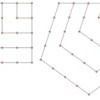Polygonals

Age 7 to 11 Challenge Level:

Polygonal numbers are those that are arranged in shapes as they enlarge. Explore the polygonal numbers drawn here.Surprising Split

Age 7 to 11 Challenge Level:

Does this 'trick' for calculating multiples of 11 always work? Why or why not?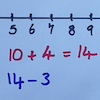Strike it Out

Age 5 to 11 Challenge Level:

Use your addition and subtraction skills, combined with some strategic thinking, to beat your partner at this game.How Odd

Age 5 to 7 Challenge Level:

This problem challenges you to find out how many odd numbers there are between pairs of numbers. Can you find a pair of numbers that has four odds between them?Round the Dice Decimals 1

Age 7 to 11 Challenge Level:

Use two dice to generate two numbers with one decimal place. What happens when you round these numbers to the nearest whole number?Magic Vs

Age 7 to 11 Challenge Level:

Can you put the numbers 1-5 in the V shape so that both 'arms' have the same total?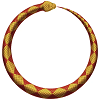Snake Coils

Age 7 to 11 Challenge Level:

This challenge asks you to imagine a snake coiling on itself.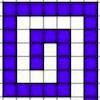Spirals, Spirals

Age 7 to 11 Challenge Level:

Here are two kinds of spirals for you to explore. What do you notice?Three Dice

Age 7 to 11 Challenge Level:

Investigate the sum of the numbers on the top and bottom faces of a line of three dice. What do you notice?Round the Dice Decimals 2

Age 7 to 11 Challenge Level:

What happens when you round these numbers to the nearest whole number?Triangle Pin-down

Age 7 to 11 Challenge Level:

Use the interactivity to investigate what kinds of triangles can be drawn on peg boards with different numbers of pegs.Domino Numbers

Age 7 to 11 Challenge Level:

Can you see why 2 by 2 could be 5? Can you predict what 2 by 10 will be?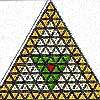The Great Tiling Count

Age 7 to 11 Challenge Level:

Compare the numbers of particular tiles in one or all of these three designs, inspired by the floor tiles of a church in Cambridge.Cut it Out

Age 7 to 11 Challenge Level:

Can you dissect an equilateral triangle into 6 smaller ones? What number of smaller equilateral triangles is it NOT possible to dissect a larger equilateral triangle into?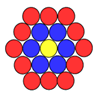Counting Counters

Age 7 to 11 Challenge Level:

Take a counter and surround it by a ring of other counters that MUST touch two others. How many are needed?Window Frames

Age 5 to 14 Challenge Level:

This task encourages you to investigate the number of edging pieces and panes in different sized windows.Dotty Circle

Age 7 to 11 Challenge Level:

Watch this film carefully. Can you find a general rule for explaining when the dot will be this same distance from the horizontal axis?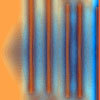Move a Match

Age 7 to 11 Challenge Level:

How can you arrange these 10 matches in four piles so that when you move one match from three of the piles into the fourth, you end up with the same arrangement?Truth or Lie

Age 7 to 11 Challenge Level:

Take a look at the video of this trick. Can you perform it yourself? Why is this maths and not magic?